# 机器学习实践

### 大纲

• 1.什么是机器学习
• a.简介
• b.有监督
• c.无监督
• 2.有监督
• a.线性回归
• b.逻辑回归
• c.神经网络
• d.sigmoid/梯度消失/relu/BN/dropout
• 3.无监督
• a.kmeans
• b.kmeans++
• c.dbscan
1. 常用工具
• a. 常用工具
• 5.预处理阶段
• a.准备数据
• b.数据清洗(归一化/缺失值/boxing..)
• c.样本划分
• 6.训练tensorflow
• a.dag简介(node/tensor/session/optimizer/)
• b.架构

### 1. 机器学习概念

#### 1.1 简介

1. 设计模型，确定查找目标函数的范围

2. 选择损失函数，制定函数的评价标准

3. 选择优化器，为机器指明如何找到目标函数的途径

#### 1.4 有监督学习（supervised learning）

• 回归（regression）预测连续的输出值• 分类（classification）预测离散的输出值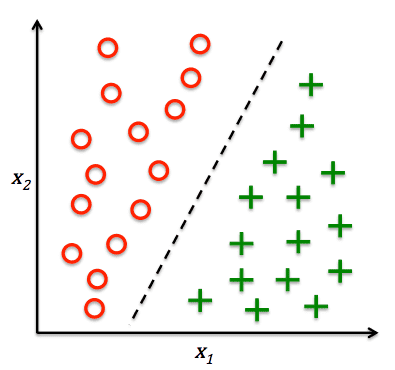#### 1.5 无监督学习（unsupervised learning）### 2. 常用算法

• 逻辑回归
• 决策树
• 随机森林算法
• SVM
• 朴素贝叶斯
• K最近邻算法
• K均值算法
• 神经网络
• 马尔可夫

#### 2.0 线性回归

y=kx+b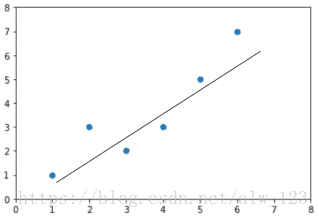#### 2.1 逻辑回归

𝑧(𝑥(𝑖))=𝜃0+𝜃1𝑥(𝑖)1+𝜃2𝑥(𝑖)2+…+𝜃𝑛𝑥(𝑖)𝑛

sigmoid=1/(1+𝑒−𝑧)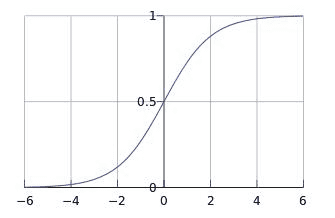m是代表m个样本，1/m 表示m个样本方差的均值，2是为了方便后面求导，其实是可以取任意实数1）首先对θ赋值，这个值可以是随机的，也可以让θ是一个全零的向量。

2）改变θ的值，使得θ按梯度下降的方向进行减少。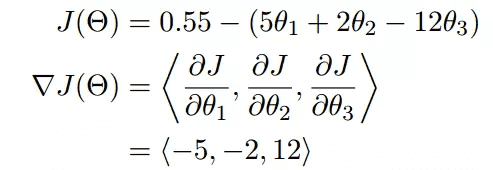#### 2.2 神经网络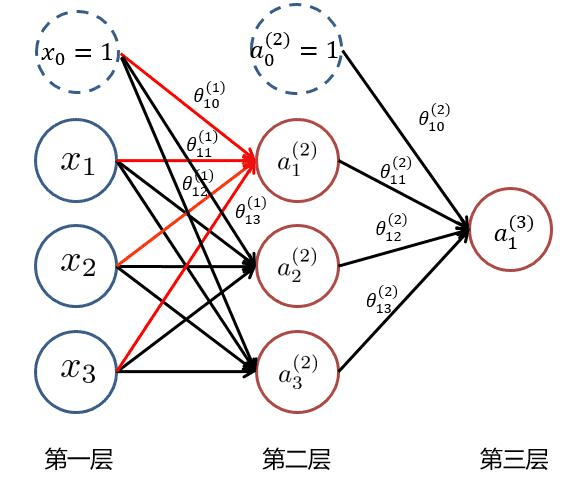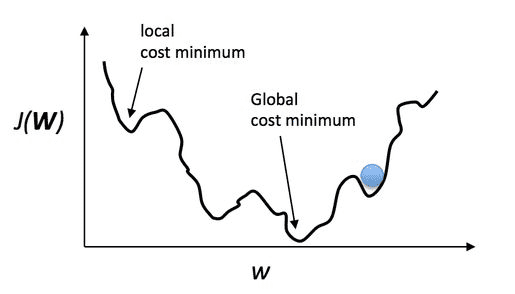#### 2.3 kmeans

Kmeans是一种无监督的聚类算法。对于给定的样本集，按照样本之间的距离大小，将样本划分为K个簇，让簇内的点尽量紧密的连接在一起，而让簇间的距离尽量的大。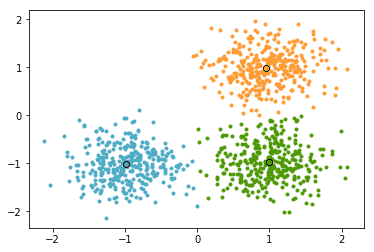### 3. 训练流程

3.1 准备数据

3.2 特征工程

• 缺失值处理
• 最简单粗暴的方法就是把含有缺失值的样本丢弃，避免人为填充带来的噪声。会丢失一些很重要的信息，特别是数据量不多或者数据价值很高的数据来说，直接丢弃就太浪费。可以使用平均值、中值、分位数、众数等替代。缺失信息也可以作为一种特殊的特征表达，如：男、女、未知。
• 连续特征离散化
• 特征可以被分为连续特征和离散特征，但有些时候我们可以会将连续特征离散化，例如，如果我们只关心年龄是否大于18，那么我们会将该特征二值化。令模型对异常数据不那么敏感。
• 归一化和标准化
• 归一化使得数据放缩在[0,1]之间并且使得特征之间的权值相同，改变了原数据的分布
• 标准化将不同特征维度的伸缩变换使得不同度量之间的特征具有可比性，不改变原始数据的分布
• 常见的归一化方法有min-max标准化和z-score标准化
• 数据变换 log1p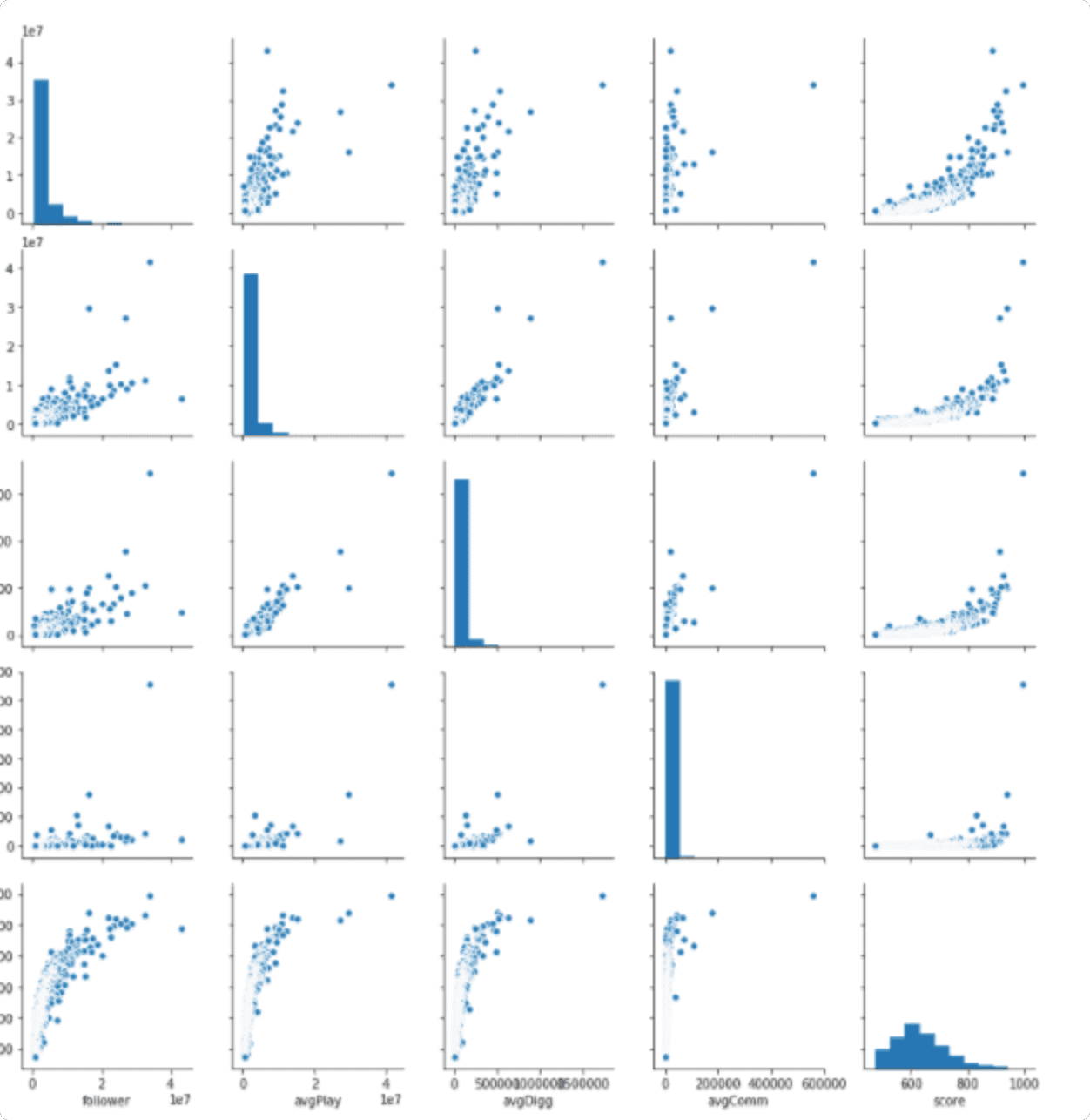3.3 baseline->tf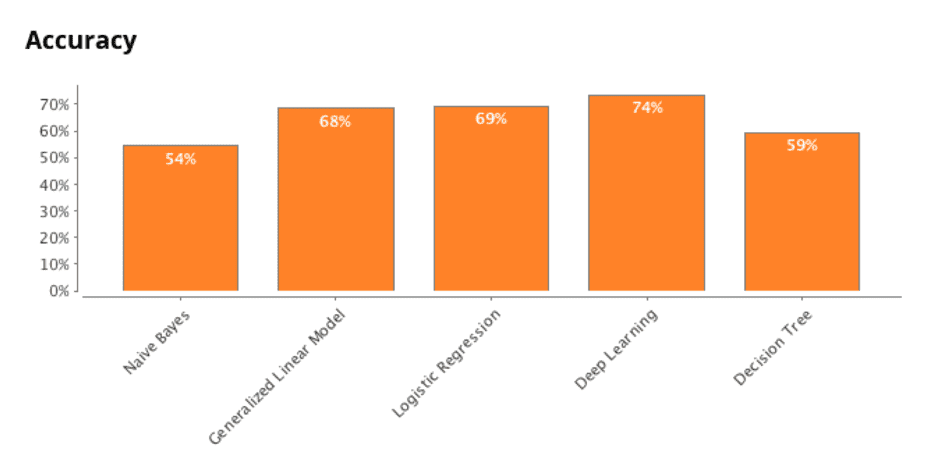3.3.5 tensorflow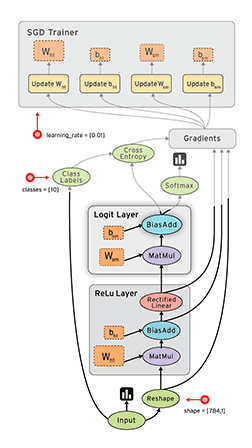3.4 调参&优化&压测

• learning_rate 尝试，过大震荡，过小速度慢

• 过拟合，dropout

• 网络大小，线上预测性能&准确率权衡

• 激活函数选择，relu

• tensorflow performance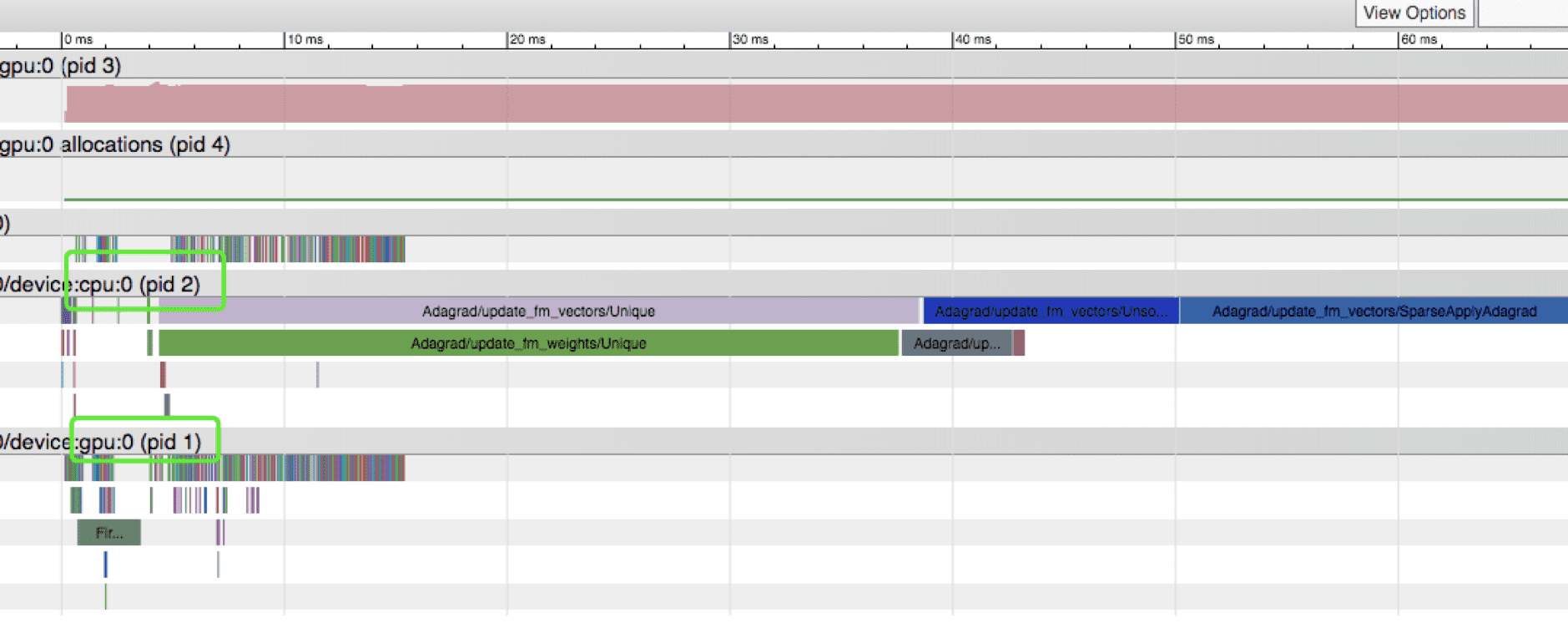### 4. 常用工具

#### 4.2 sklearn(scikit-learn)

Scikit-learn(sklearn)是机器学习中常用的第三方模块，对常用的机器学习方法进行了封装，包括回归(Regression)、降维(Dimensionality Reduction)、分类(Classfication)、聚类(Clustering)等方法。

#### 4.3 numpy

NumPy(Numerical Python) 是 Python 语言的一个扩展程序库，支持大量的维度数组与矩阵运算，此外也针对数组运算提供大量的数学函数库。

#### 4.4 matplotlib

Matplotlib是一个Python 2D绘图库，生成绘图，直方图，功率谱，条形图，错误图，散点图等。

``````import numpy as np
import matplotlib.pyplot as plt
from sklearn.cluster import KMeans
from sklearn.datasets import make_blobs

X, y = make_blobs(n_samples=1500, random_state=170)
y_pred = KMeans(n_clusters=2, random_state=random_state).fit_predict(X)

plt.subplot(221)
plt.scatter(X[:, 0], X[:, 1], c=y_pred)
plt.title("Incorrect Number of Blobs")
``````#### 4.5 pandas

``````import numpy as np
import pandas as pd

movie_ratings = pd.merge(df_ratings, df_items)
lens = pd.merge(movie_ratings, df_user)

scientist_ratings = lens.query("occupation == 'scientist'")
top_scientist_ratings = scientist_ratings.groupby('movie_title').agg({'rating': [np.size, np.mean]})

top_5_percentile = np.percentile(top_scientist_ratings[('rating','size')], 95.0)
top_scientist_ratings = top_scientist_ratings[top_scientist_ratings[('rating','size')] > top_5_percentile]
top_scientist_ratings.sort_values([('rating', 'mean')], ascending=False)
``````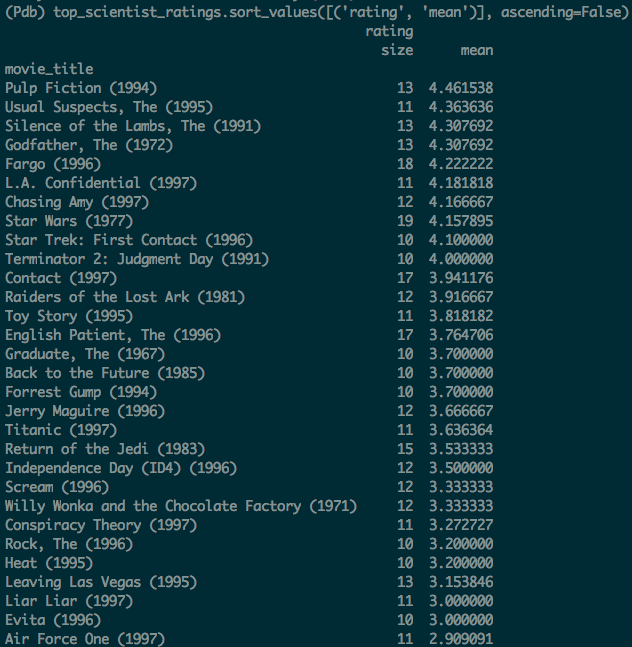#### 4.6 tensorflow

TensorFlow是一个采用数据流图（data flow graphs），用于数值计算的开源软件库。常用来做神经网络相关的机器学习任务。

#### 4.7 keras

Keras是一个高层神经网络API，Keras由纯Python编写而成并基Tensorflow、Theano以及CNTK后端。Keras 为支持快速实验而生，能够把你的idea迅速转换为结果。

#### 4.8 Others

sklearn_pandas、gensim…